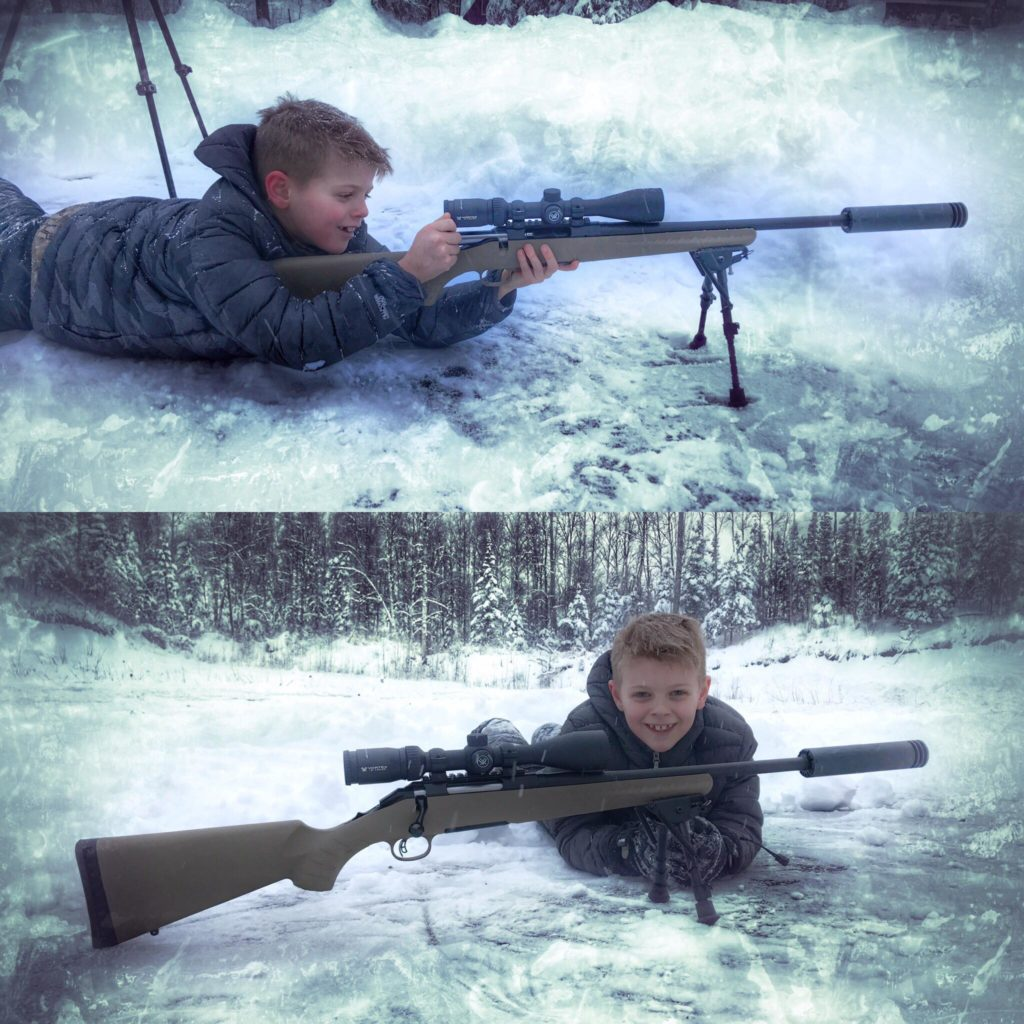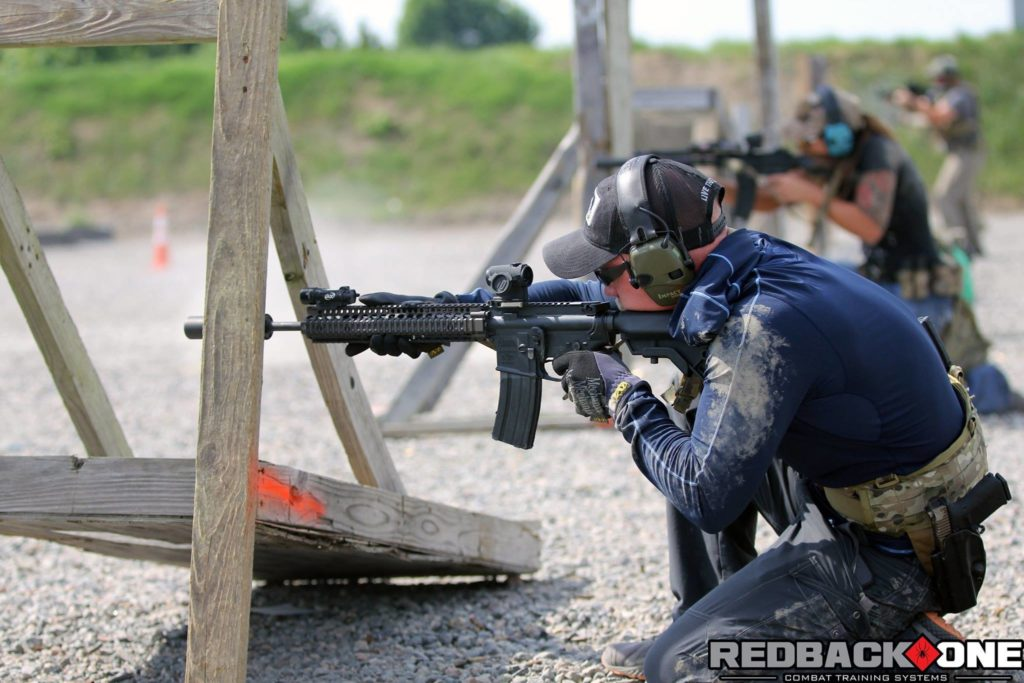# Rifle Recoil - Why the Pain?

#### Rifle Recoil - Why the Pain?!

Welcome to Rifle Recoil 101.  Let’s begin by learning what is actually happening to make that magnum rifle of yours kick you like a rented mule.  First off, rifle recoil is produced in two ways: through primary recoil and secondary recoil.  We will tackle primary recoil first, which is caused by the weight of the bullet as it accelerates down the barrel.  To explain what's going on after your firing pin strikes the primer, you're going to have to buckle up, as we're taking a trip back to your high school physics classroom.

Before we start, I'll show you a quick video of a friend of mine shooting what he refers to as being the hardest recoiling rifle he has ever fired.  No, we haven't had a chance to dive into the heavy double rifle and the like being toted around the dark continent, but this .338 RUM weighing in at 6.5 pounds wearing just iron sights produced enough rifle recoil for him to turn down the offer of a crisp \$100 bill if he would've shot it prone.  What are friends for, right?!

The physics principles at play here are very simple and ones that we are likely very familiar with.  We are going to explain this in a step-by-step format which will certainly minimize the size of your headache after reading this (hopefully).

Most of us have been exposed to Sir Isaac Newtons Laws of Motion at some point during our schooling and we are going to look at each one as all are relevant to this topic.

Newtons First Law states that an object at rest will remain at rest unless acted upon by an outside force.  Also, an object in motion continues in motion with the same speed and in the same direction unless acted upon by an outside force.  This law is often called "the law of inertia".  More simply, this means that the gun is not going to move by itself or counter recoil by itself.  The gun will not move or exert force (on your poor shoulder) until a force is exerted upon it.  This is easy to envision, and also applies to pistols as well.  You can see the recoil being exerted on the pistol in the video below and the slide reciprocating as the force is applied to it.  Well, that was easy, let’s keep going!

Newtons Second Law states that acceleration is produced when a force acts on a mass.  The greater the mass (of the object being accelerated), the greater the amount of force needed to accelerate the object (bullet in our case).  Everyone inherently knows that heavier objects require more force to move the same distance as lighter objects.  The acceleration of our bullets is directly proportional to the amount of force (burning powder) being applied to them and inversely proportional to the mass of the bullet.  This means that how fast your gun and bullet accelerate are going to depend directly on the amount of force applied to it, and the weight of the gun or bullet.  We now see a clear relationship between force, mass, and acceleration.  It is familiarly expressed as Force = Mass x Acceleration, or F=ma.

Taking that equation, you can now calculate how much force is generated from an object with a known weight accelerating at a known rate.  Known rate, known weight, you got your force!  "What is acceleration?" you ask?  Acceleration (a) is basically the change in velocity (v) over a given time (t).  Our F=ma equation will give you the amount of force being applied during the acceleration of our bullet.  After our bullet has stopped accelerating, you can calculate the amount of kinetic energy imparted to it through its acceleration by using the equation KE=mv^2.

You can do this because the "a" in our F=ma equation has gone to zero after the bullet has stopped accelerating!  The kinetic energy (KE) is going to determine how powerful your bullet is while the F=ma equation will determine how much recoil it is going to generate getting up to its maximum speed through its acceleration process.  Keep this in the back of your head as we will discuss it in the next section.Adding a silencer to the rifle not only adds weight, but acts as a large muzzle brake, reducing both primary and secondary recoil!

Lastly, Newtons third law states that for every action, there is an equal and opposite reaction.  This basically tells us that if you want to push heavy bullets at lightning speeds from your barrel, you're going to pay for it on the butt pad of the rifle!  As the bullet accelerates forward, the gun HAS to accelerate rearward.  No free lunch here, guys.  Since these forces are equal, the equations can look something like this:

F=ma(gun) = F=ma(bullet)

Since the forces are equal, taking the difference of these will always give you zero.  This explains the reason that if the new hand load you worked up has your bullets going faster or your rifle goes on a diet, this translates into a healthy dose of increased rifle recoil because the rifles acceleration will increase.

Instead of talking it to death, let's put some simple numbers into these equations so they start to mean something.  There are 7000 grains in a pound, so if your rifle weighs 7 pounds, you have a 49,000 grain rifle.  I know, I know, that sounds weird, but let’s stick with it.  Let’s also say you are shooting a 150 grain bullet out of your .30 caliber rifle.  For simplicities sake we will ignore the acceleration constants, exponents and whatnot, and just give the bullet an acceleration value of 100 since we haven't applied specific units of measurement and are just in search of relative values.

Sticking with our equal force equation we will see that 49,000(a) = 150(a).  Plug in our acceleration value for the bullet and we see that our values are 49,000(a) = 15,000.  Dividing these numbers to solve for 'a', you see that the acceleration of the rifle is .00306 or 3.06% the acceleration of the bullet, proving our statement that changing the weight or acceleration of the bullet is going to change the acceleration (recoil) of the rifle.

Like I stated above, that covered what primary rifle recoil is and how it is calculated.  Secondary rifle recoil is caused by the propulsion gasses as they blast out of the muzzle.  Like primary rifle recoil, they propel the rifle backward into your shoulder, adding insult to the injury of your already bruised shoulder.  Once that pesky bullet leaves the barrel and gets out of the way, it frees up the propulsion gasses to suddenly accelerate a lot faster in the forward direction, creating more recoil force in the rearward direction.  Since the forward acceleration forces will always equal the rearward acceleration forces, we can reasonably calculate how much these additional factors are going to add to the rifle recoil.  We will make a very conservative estimation and say that the propellant is going to make a 25% acceleration rate increase once the bullet leaves the barrel but for only 25% of the previous time, therefore we will list that force separately in the equation to keep things simplified.No matter the size of the cartridge fired, the physics remains the same

Let’s account for the forces.  Don’t let this equation scare you right off the bat.  It’s the same as before, just adding the force of the initial propellant recoil and the force of the secondary propellant recoil.

ma(rifle)=ma(bullet)+ma(propellant)+.25m(1.25)a {propellant second}

Sticking with our 150 grain bullet, we will add a 50 grain charge of powder.  Now we have an equation that looks like this:

49,000(a)=15,000+5,000+1562.5

or

49,000(a)=21,562.5

Solving for the acceleration of the rifle again, we find that the rifle now has 4.4% the acceleration rate of the bullets acceleration rate.  You say "Big deal, you increased the acceleration of the rifle by 1.34%".  You are correct, but look at it from the perspective of increasing the rearward acceleration of the rifle by 43.8%!  That’s a SUBSTANTIAL increase in recoil over what would be created by accelerating the bullet alone.

So the next time you bring the Holland brothers to the range and chamber a freshly loaded 375 H&H in your hunting rifle, remember this article, then pull the trigger.